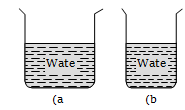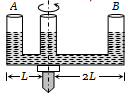The density $\mathrm{\rho }$ of water of bulk modulus B at a depth y in the ocean is related to the density at surface ${\mathrm{\rho }}_{0}$ by the relation

(a) $\mathrm{\rho }={\mathrm{\rho }}_{0}\left[1-\frac{{\mathrm{\rho }}_{0}\mathrm{gy}}{\mathrm{B}}\right]$                            (b) $\mathrm{\rho }={\mathrm{\rho }}_{0}\left[1+\frac{{\mathrm{\rho }}_{0}\mathrm{gy}}{\mathrm{B}}\right]$

(c) $\mathrm{\rho }={\mathrm{\rho }}_{0}\left[1+\frac{\mathrm{B}}{{\mathrm{\rho }}_{0}\mathrm{gy}}\right]$                             (d) $\mathrm{\rho }={\mathrm{\rho }}_{0}\left[1-\frac{\mathrm{B}}{{\mathrm{\rho }}_{0}\mathrm{gy}}\right]$

High Yielding Test Series + Question Bank - NEET 2020

Difficulty Level:

With rise in temperature, density of a given body changes according to one of the following relations

(a)                         (b)

(c)                                 (d)

Concept Questions :-

Properties of fluids
High Yielding Test Series + Question Bank - NEET 2020

Difficulty Level:

From the adjacent figure, the correct observation is(a) The pressure on the bottom of tank (a) is greater than at the bottom of (b).
(b) The pressure on the bottom of the tank (a) is smaller than at the bottom of (b)
(c) The pressure depend on the shape of the container
(d) The pressure on the bottom of (a) and (b) is the same

Concept Questions :-

Pressure
High Yielding Test Series + Question Bank - NEET 2020

Difficulty Level:

A given shaped glass tube having uniform cross section is filled with water and is mounted on a rotatable shaft as shown in figure. If the tube is rotated with a constant angular velocity $\mathrm{\omega }$ then(a) Water levels in both sections A and B go up
(b) Water level in Section A goes up and that in B comes down
(c) Water level in Section A comes down and that in B it goes up
(d) Water levels remains same in both sections

Concept Questions :-

Pressure

Difficulty Level:

An ice berg of density 900 Kg/${\mathrm{m}}^{3}$ is floating in water of density 1000 Kg/${\mathrm{m}}^{3}$. The percentage of volume of ice-cube outside the water is
(a) 20%                                       (b) 35%
(c) 10%                                       (d) 25%

Concept Questions :-

Archimedes principle
High Yielding Test Series + Question Bank - NEET 2020

Difficulty Level:

A log of wood of mass 120 Kg floats in water. The weight that can be put on the raft to make it just sink, should be (density of wood = 600 Kg/${\mathrm{m}}^{3}$
(a) 80 Kg                                                 (b) 50 Kg
(c) 60 Kg                                                  (d) 30 Kg

Concept Questions :-

Archimedes principle
High Yielding Test Series + Question Bank - NEET 2020

Difficulty Level:

A hemispherical bowl just floats without sinking in a liquid of density . If outer diameter and the density of the bowl are 1 m and  respectively, then the inner diameter of the bowl will be

(a) 0.94 m                            (b) 0.97 m
(c) 0.98 m                            (d) 0.99 m

Concept Questions :-

Archimedes principle
High Yielding Test Series + Question Bank - NEET 2020

Difficulty Level:

In making an alloy, a substance of specific gravity ${\mathrm{s}}_{1}$ and mass ${\mathrm{m}}_{1}$ is mixed with another substance of specific gravity ${\mathrm{s}}_{2}$ and mass ${\mathrm{m}}_{2}$ ; then the specific gravity of the alloy is

1. $\left(\frac{{\mathrm{m}}_{1}+{\mathrm{m}}_{2}}{{\mathrm{s}}_{1}+{\mathrm{s}}_{2}}\right)$                      2. $\left(\frac{{\mathrm{s}}_{1}{\mathrm{s}}_{2}}{{\mathrm{m}}_{1}+{\mathrm{m}}_{2}}\right)$

3. $\frac{{\mathrm{m}}_{1}+{\mathrm{m}}_{2}}{\left(\frac{{\mathrm{m}}_{1}}{{s}_{1}}+\frac{{m}_{2}}{{\mathrm{s}}_{2}}\right)}$                  4. $\frac{\left(\frac{{\mathrm{m}}_{1}}{{\mathrm{s}}_{1}}+\frac{{\mathrm{m}}_{2}}{{\mathrm{s}}_{2}}\right)}{{\mathrm{m}}_{1}+{\mathrm{m}}_{2}}$

Concept Questions :-

Properties of fluids
High Yielding Test Series + Question Bank - NEET 2020

Difficulty Level:

A concrete sphere of radius R has a cavity of radius r which is packed with sawdust. The specific gravities of concrete and sawdust are respectively 2.4 and 0.3 for this sphere to float with its entire volume submerged under water. Ratio of mass of concrete to mass of sawdust will be
(a) 8                                        (b) 4
(c) 3                                        (d) Zero

Concept Questions :-

Properties of fluids
High Yielding Test Series + Question Bank - NEET 2020

Difficulty Level:

A metallic block of density 5 gm ${\mathrm{cm}}^{-3}$ and having dimensions 5 cm × 5 cm × 5 cm is weighed in water. Its apparent weight will be
(a) 5 × 5 × 5 × 5 gf                            (b) 4 × 4 × 4 × 4 gf
(c) 5 × 4 × 4 × 4 gf                            (d) 4 × 5 × 5 × 5 gf

Concept Questions :-

Archimedes principle